﻿ JS中位运算符的一些妙用 - 自由资讯

# JS中位运算符的一些妙用

##### 目录
• 前言：
• 1. 使用左移运算符 << 迅速得出2的次方
• 2. 使用 ^ 切换变量 0 或 1
• 3. 使用 & 判断奇偶性
• 4. 使用 !! 将数字转为布尔值
• 5. 使用~、>>、<<、>>>、|来取整
• 6. 使用^来完成值交换
• 7. 使用^判断符号是否相同
• 8. 使用^来检查数字是否不相等
• 9. n & (n - 1)，如果为 0，说明 n 是 2 的整数幂
• 10. 使用 A + 0.5 | 0 来替代 Math.round()
• String
• 1. 使用toString(16)取随机字符串
• 2. 使用 split(0)
• 4. 使用 Array 来重复字符
• 其他一些花里胡哨的操作
• 1. 使用当前时间创建一个随机数
• 2. 一些可以替代 undefined 的操作
• 3. 使用 Array.length = 0 来清空数组
• 4. 使用 Array.slice(0) 实现数组浅拷贝
• 5. 使用 !+“1” 快速判断 IE8 以下的浏览器
• 6. for 循环条件的简写
• 总结

## 前言：

Javascript 完全套用了 Java 的位运算符，包括按位与&、按位或|、按位异或^、按位非~、左移<<、带符号的右移>>和用0补足的右移>>>。这套运算符针对的是整数，所以对 JavaScript 完全无用，因为 JavaScript 内部，所有数字都保存为双精度浮点数。如果使用它们的话，JavaScript 不得不将运算数先转为整数，然后再进行运算，这样就降低了速度。而且"按位与运算符"&同"逻辑与运算符"&&，很容易混淆。

## 1. 使用左移运算符 << 迅速得出2的次方

```	1 << 2  // 4, 即 2的2次方
1 << 10 // 1024, 即 2的10次方

// 但是要注意使用场景
a = 2e9;   // 2000000000
a << 1;    // -294967296
```

## 2. 使用 ^ 切换变量 0 或 1

```	// --- before ---
// if 判断
if (toggle) {
toggle = 0;
} else {
toggle = 1;
}
// 三目运算符
togle = toggle ? 0 : 1;

// --- after ---
toggle ^= 1;
```

## 3. 使用 & 判断奇偶性

```	console.log(7 & 1);    // 1
console.log(8 & 1) ;   // 0
```

## 4. 使用 !! 将数字转为布尔值

所有非0的值都是true，包括负数、浮点数：

```	console.log(!!7);       // true
console.log(!!0);       // false
console.log(!!-1);      // true
console.log(!!0.71);    // true
```

## 5. 使用~、>>、<<、>>>、|来取整

相当于使用了 Math.floor()

```	console.log(~~11.71)     // 11
console.log(11.71 >> 0)  // 11
console.log(11.71 << 0)  // 11
console.log(11.71 | 0)   // 11
console.log(11.71 >>> 0) // 11
```

注意 >>> 不可对负数取整

## 6. 使用^来完成值交换

在 ES6 的解构赋值出来之前，用这种方式会更快(但必须是整数)：

```	// --- before ---
let temp = a; a = b; b = temp; // 传统，但需要借助临时变量
b = [a, a = b] // 借助数组

// --- after --- 只能用于整数
let a = 7
let b = 1
a ^= b
b ^= a
a ^= b
console.log(a)   // 1
console.log(b)   // 7

[a, b] = [b, a]; // ES6，解构赋值
```

## 7. 使用^判断符号是否相同

只能应用与整数

```	(a ^ b) >= 0; //  true 相同; false 不相同
```

## 8. 使用^来检查数字是否不相等

```	// --- before ---
if (a !== 1171) {...};

// --- after ---
if (a ^ 1171) {...};
```

## 9. n & (n - 1)，如果为 0，说明 n 是 2 的整数幂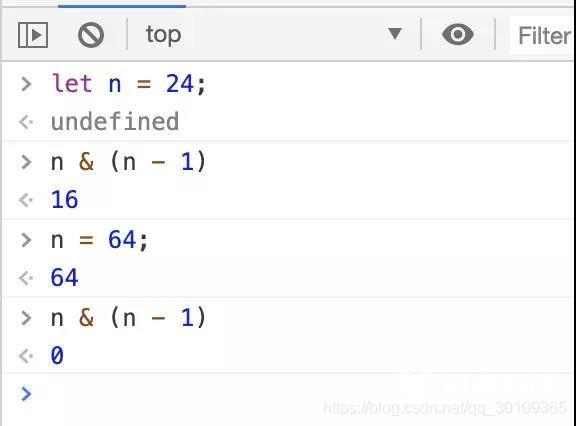## 10. 使用 A + 0.5 | 0 来替代 Math.round()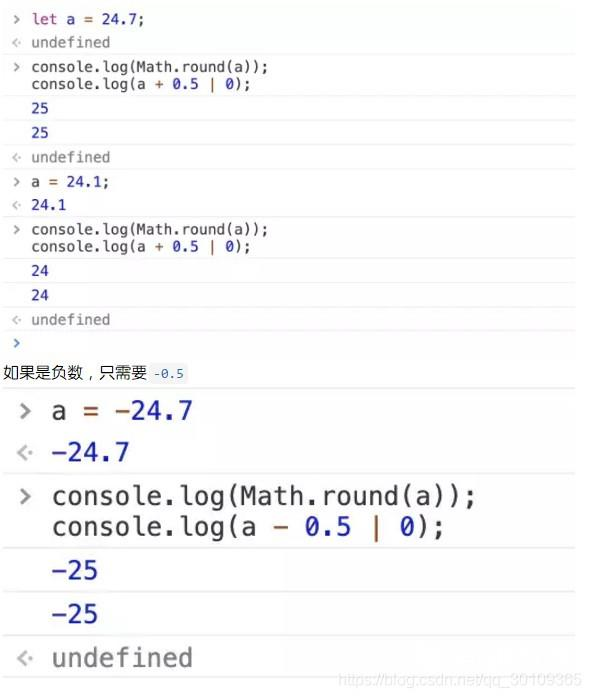## String

### 1. 使用toString(16)取随机字符串

```	Math.random().toString(16).substring(2, 15);
```

.substring() 的第二个参数控制取多少位 (最多可取13位)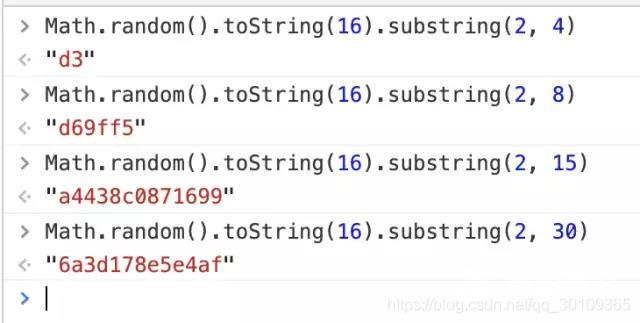### 2. 使用 split(0)

使用数字来做为 split 的分隔条件可以节省2字节（不实用）

```	// --- before ---
"alpha,bravo,charlie".split(",");

// --- after ---
"alpha0bravo0charlie".split(0);
```

一个鲜为人知的方法，可以快速创建 a 标签

```	// --- before ---

// --- after ---
```

### 4. 使用 Array 来重复字符

```    // --- before ---
for (let a = "", i = 7; i--;) a+= 0;

// --- after ---
let b = Array(7).join(0); // "0000000"let c = Array(7).join( La ) // "LaLaLaLaLaLa"

// ES6
let d = "0".repeat(7); // "0000000"
```

## 其他一些花里胡哨的操作

### 1. 使用当前时间创建一个随机数

```    // --- before ---
let b = 0 | Math.random() * 100

// --- after ---
let a;
a = new Date % 100; // 两位随机数
a = new Date % 1000; // 三位随机数
a = new Date % 10000; // 四位随机数...依次类推
// 不要在快速循环中使用，因为毫秒可能没有变化；
```

### 2. 一些可以替代 undefined 的操作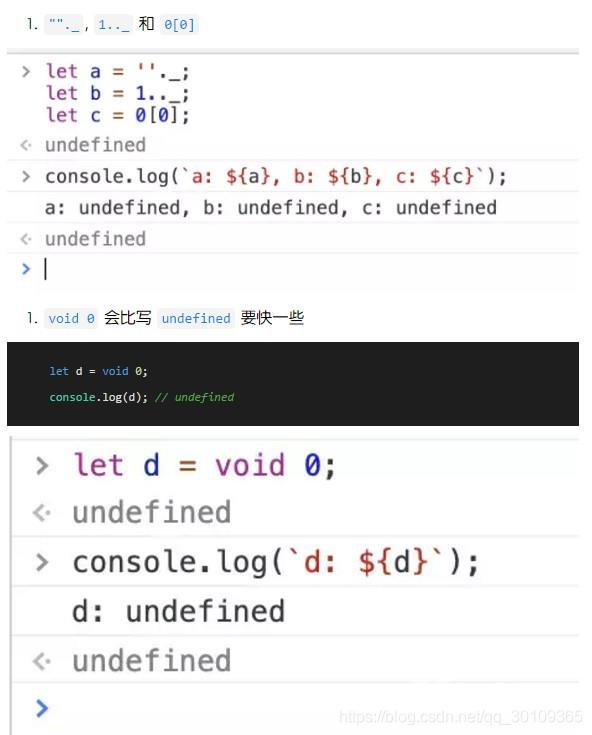### 5. 使用 !+“1” 快速判断 IE8 以下的浏览器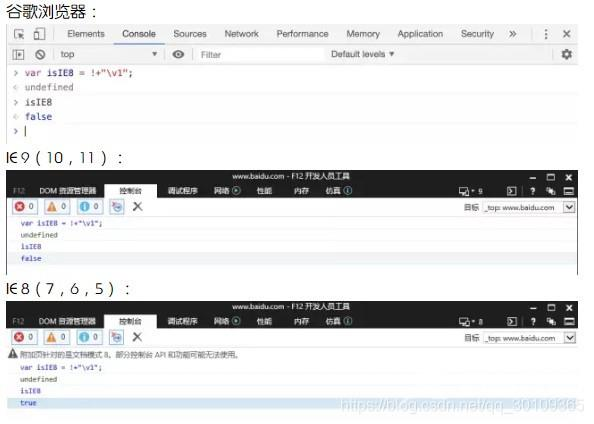### 6. for 循环条件的简写

```    // --- before ---
for(let i = 0; i < arr.length; i++) {...}

// --- after ---
for(let i = arr.length; i--;) {...} // 注意 i-- 后面的分号别漏了
```

## 总结

到此这篇关于JS中位运算符的一些妙用的文章就介绍到这了,更多相关JS位运算符妙用内容请搜索自由互联以前的文章或继续浏览下面的相关文章希望大家以后多多支持自由互联！

上一篇：js金额千分位的6种实现方法实例
下一篇：没有了
网友评论
相关栏目
﻿